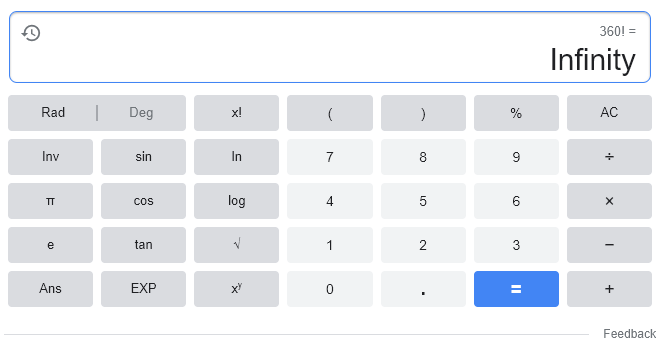# Poisson Distribution

Let's explore the appearance of various Poisson distributions by modifying the parameter λ.
What does the value k on the x-axis of the Poisson distribution represent?
Can you explain what the length of each bar represents?

The Poisson distribution is more useful in real life than you might think, just like the binomial distribution.

Despite its complex name and difficult-looking formula, it can become a friendlier companion than the normal distribution once you understand what it actually means.

# Prerequisites

To understand the Poisson distribution well, it is recommended to know the following:

# Definition of Poisson distribution

If we denote the expected value of the number of events that occur within a fixed time interval by $\lambda$, the probability that the event occurs $n$ times is as follows:

$f(n;\lambda) = \frac{\lambda^n e^{-\lambda}}{n!}$

Here, $e$ is the natural constant.

# Why do we need the Poisson distribution?

Let’s imagine looking out the window for an hour.

There are many people passing by outside, but what is the likelihood that one of them will trip and fall?

Suppose 1,000 people are passing by, and assume that one person falls down every 10 seconds, which means that there is a 0.1% chance of someone falling down every 10 seconds.

Then, we can consider that we are assuming that the events that occur independently with a 0.1% chance every 10 seconds occur 360 times in a 3,600-second period.

In such cases, we can use the binomial distribution to determine the probability distribution of the number of people who fall during the hour.

In other words, let’s solve a problem assuming that it follows the binomial distribution $B(n,p)$ with the total number of trials $n$ and the probability of success $p$. Although it is easy to conclude that on average, it will be 0.36 people, which is $360 \times 0.001$, can we easily calculate its distribution?

In other words, the probability of an event occurring $k$ times out of 360 is:

$B(n, p;k) = B(360, 0.001; k) = \frac{360!}{k!(360-k)!}(0.001)^k (0.999)^{360-k}$

You can intuitively understand that 360 factorial is an immensely large number that is practically infinite.

In fact, if you try to calculate 360 factorial on Google, it will say ‘impossible’.Figure 1. Factorials of extremely large numbers are impossible to calculate.

Therefore, it is desirable to propose a new form of distribution using a limit value to approximately calculate the probability distribution of the binomial distribution when $n$ is too large and $p$ is too small.

The Poisson distribution can be said to have been born as a distribution to solve this problem.

# Derivation of the Poisson distribution

The Poisson distribution can be derived from the binomial distribution.

If we let $\lambda = np$, then $p=\frac{\lambda}{n}$, so

$Pr(K=k) = {}_n\mathrm{ C }_kp^k(1-p)^{n-k}$ $=\frac{n(n-1)\cdots(n-k+1)}{k!}\left(\frac{\lambda}{n}\right)^k\left(1-\frac{\lambda}{n}\right)^n\left(1-\frac{\lambda}{n}\right)^{-k}$ $=\left(\frac{\lambda^k}{k!}\right)\frac{n(n-1)\cdots(n-k+1)}{n^k}\left(1-\frac{\lambda}{n}\right)^n\left(1-\frac{\lambda}{n}\right)^{-k}$

Taking the limit as $n\rightarrow \infty$, we can see the following changes occur.

$\lim_{n\rightarrow \infty}p=\lim_{n\rightarrow \infty}\frac{\lambda}{n} = 0$ $\lim_{n\rightarrow \infty}\frac{n(n-1)\cdots(n-k+1)}{n^k} = 1$ $\lim_{n\rightarrow \infty}\left(1-\frac{\lambda}{n}\right)^n =\lim_{n\rightarrow \infty}\left(1-\frac{\lambda}{n}\right)^{-\frac{n}{\lambda} \times (-\lambda)} = e^{-\lambda}$ $\lim_{n\rightarrow \infty}\left(1-\frac{\lambda}{n}\right)^k=1$

Therefore, it can be known that

$Pr(K=k)\rightarrow \left(\frac{\lambda^k}{k!}\right)e^{-\lambda}$

In other words, the Poisson distribution is a distribution of a probability variable that has a very low probability of a specific event occurring among many events (i.e., $n\rightarrow \infty$) and has a small probability (i.e., $p\rightarrow 0$).

# Example Problem of Poisson Distribution

## Problem 1

Insurance company A has 2,000 insurance contracts. Assuming the probability of a person who signed the insurance contract making a claim is 0.001, use the Poisson approximation to find the following probabilities.

1) The probability that no claims will be made.

2) The probability that at most 3 claims will be made.

3) The probability that 5 or more claims will be made.

### Solution to Problem 1

Since the probability of a claim being made for each of the 2,000 insurances is 0.001, it can be known that the expected number of claims is 2.

1) The probability that no claims will be made is when $k=0$ in the Poisson distribution with $\lambda=2$:

$Pr(K=0) = \left(\frac{2^0}{0!}\right)e^{-2} = 0.13534$

Therefore, the probability is approximately 13.5%.

2) The probability that at most 3 claims will be made is:

$\sum_{i=0}^3Pr(K=i) = \left(\frac{2^0\times e^{-0}}{0!}+\frac{2^1\times e^{-1}}{1!}+\frac{2^2\times e^{-2}}{2!}\right)=0.85712$

Therefore, the probability is approximately 85.7%.

3) The probability of having 5 or more insurance claims is given by:

$1-\sum_{i=0}^4Pr(K=i)=1-\left( \frac{2^0\times e^{-0}}{0!}+ \frac{2^1\times e^{-1}}{1!}+ \frac{2^2\times e^{-2}}{2!}+ \frac{2^3\times e^{-3}}{3!}+ \frac{2^4\times e^{-4}}{4!}\right) = 0.052653$

In other words, we can see that it is about 5.26%. This means that the probability of having 5 or more insurance claims is quite low, around 5%.

## Problem 2.

A health center in a remote area is not very busy as patients rarely visit. If the average number of patients per day is 3, what is the probability that two or more patients will visit in a day?

### Solution to Problem 2

To calculate the probability of two or more patients visiting the health center, we need to add up all the cases where there are 2 patients, 3 patients, and so on up to an infinite number of patients, and then subtract this from 1, since the total probability must equal 1.

That is,

$Pr(K>=2)=1-\sum_{i=0}^1Pr(K=i)=1-\left(\frac{e^{-3}\times 3^0}{0!}+\frac{e^{-3}\times 3^1}{1!}\right)$ $= 1-(0.0498+0.1494) = 0.8008$

Therefore, there is about an 80% chance of having two or more patients visit the health center in a day.